### Neukirch

Posted on June 1, 2019

Here are my exercise solutions and notes for Neukirch’s Algebraic Number Theory.

1.1. Recall that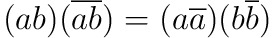for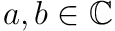. Therefore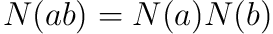for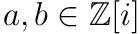. Moreover,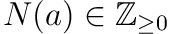for all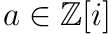. Thus if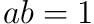we must have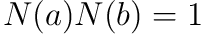and therefore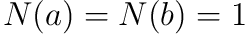.

1.2. Suppose that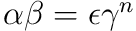for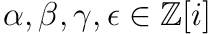with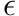a unit and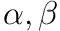relatively prime. Since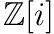is a UFD we may write out the prime factorizations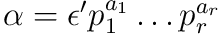and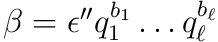with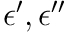units and the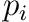’s and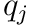’s prime. Sinceare relatively prime, the’s and’s are all distinct primes. Therefore, since, we must have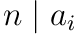and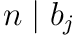for all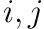. I.e., we have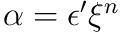and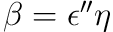where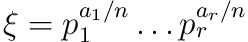and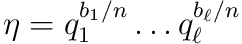.

1.3. Observe that if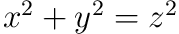for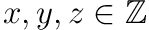, then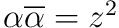for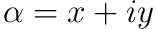. Therefore by Exercise 1.2 we have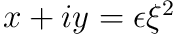witha unit and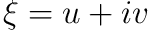. Therefore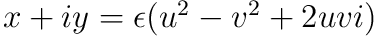. So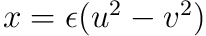and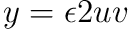. From Exercise 1 we see that the only units inare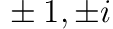. We cannot have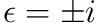as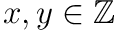. Thus we may ignore theas it just swaps the sign.

Observe that if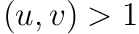then we we would have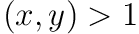, a contradiction. If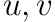are both odd, then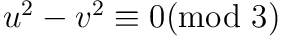and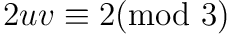. Thus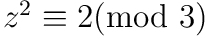, a contradiction.

1.4. Recall that an ordering on a ring satisfies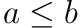implies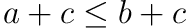for all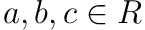, as well as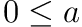and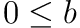implies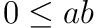for all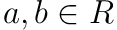. The first implication shows that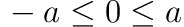for all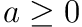; in particular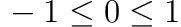. If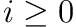, then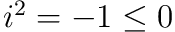, a contradiction. However, if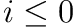then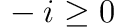and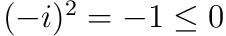.

1.5. Observe that we may define a norm on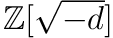by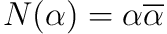where if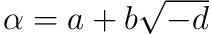we have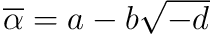. This gives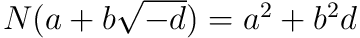. Again we see that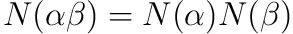and thus an element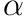is a unit if and only if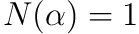. The only elements ofwith norm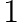are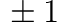.

1.6. After struggling for a while and digging a bit on math stack exchange, it appears that this question is fairly difficult for its place in the book. Perhaps I will revisit this question after progressing further.